THE LAWS OF SINES AND COSINES

Example 1:

For the oblique triangle with measures a = 12 ft, b = 31 ft, and A = 20.5o, find the remaining sides and angles. Round all calculations to two decimal places.

Assume angles A, B, and C are opposite sides labeled a, b, and c respectively.

HINT: Make a sketch!!!Given two sides and one angle opposite one of the sides, we can use the Law of Sines.

Solve for Angle B:

Specifically, we'll write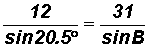12 sin B = 31 sin 20.5o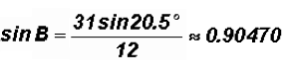Please note that sin B is positive. We know that the value of sine is positive for QI and QII angles!

NOTE: Since the sum of interior angles in a triangle is 180o and QI and QII angles are less than 180o, we could get two solutions for angle B, one acute and one obtuse angle.

Using the calculator, we find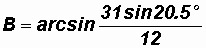(Here we used the exact value and NOT 0.90470!)

Then, the QI angle is B164.78o.

Since B1 is also the reference angle, we find the QII angle to be

B2180o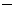64.78o = 115.22o

Both of these angles could be solutions for the given triangle! As long as angle C is not negative, we will have a triangle with two solution sets.

Solve for Angle C:

We know that A = 20.5o, B164.78o, and B2115.22o.

Thenand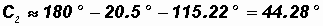Since both measures for angle C are positive the triangle indeed has TWO solution sets.

Solve for Side c:

Given, let's find sideusing the Law of Sines since we have two sides and one opposite angle: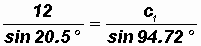12 sin 94.72o = c1 sin 20.5o

DO NOT CALCULATE the individual values of the trigonometric ratios, instead use the EXACT value as shown below.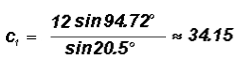Given, let's find sideby using the Law of Sines.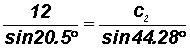12 sin 44.28o = c2 sin 20.5o

DO NOT CALCULATE the individual values of the trigonometric ratios, instead use the EXACT value as shown below.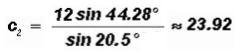The triangle has the following solutions:

Given A = 20.5o, a = 12 ft, and b = 31 ft,

we found B164.78o, C194.72o, and c134.15 ft

or B2115.22o, C244.28o, and c223.92 ft

Example 2:

For the oblique triangle with measures a = 22 in, b = 12 in, and A = 42o, find the remaining sides and angles. Round all calculations to two decimal places.

Assume angles A, B, and C are opposite sides labeled a, b, and c respectively.

HINT: Make a sketch!!!Given two sides and one angle opposite one of the sides, we can use the Law of Sines.

Solve for Angle B:

Specifically, we'll write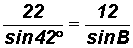22 sin B = 12 sin 42o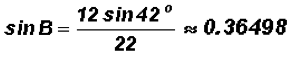Please note that sin B is positive. We know that the value of sine is positive for QI and QII angles!

NOTE: Since the sum of interior angles in a triangle is 180o and QI and QII angles are less than 180o, we could get two solutions for angle B, one acute and one obtuse angle.

Using the calculator, we find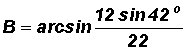(Here we used the exact value and NOT 0.36498!)

Then, the QI angle is B121.41o.

Since B1 is also the reference angle, we find the second-quadrant angle to be

B2180o21.41o = 158.59o

Both of these angles could be solutions for the given triangle! As long as angle C is not negative, we will have a triangle with two solution sets.

Solve for Angle C:

We know that A = 42o, B121.41o, and B2158.59o.This is NOT a solution, since a triangle has NO negative interior angles. Thus,is also NOT a solution.

We have just proven that this particular triangle only has ONE solution set!

Solve for Side c:

Given C116.59o , let's find side c using the Law of Sines since we have two sides and one opposite angle:DO NOT CALCULATE the individual values of the trigonometric ratios, instead use the EXACT value as shown below.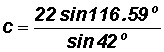c29.40 in

The triangle has the following solutions:

Given A = 42o, a = 22 in, and b = 21 in,

we found B21.41o, C116.59o, and c29.40 in

Example 3:

For the oblique triangle with measures a = 15 inches, b = 25 inches, and A = 85o, find the remaining sides and angles. Round all calculations to two decimal places.

Assume angles A, B, and C are opposite sides labeled a, b, and c respectively.

HINT: Make a sketch!!!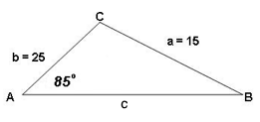Given two sides and one angle opposite one of the sides, we can use the Law of Sines.

Solve for Angle B:

Specifically, we'll write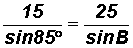15 sin B = 25 sin 85o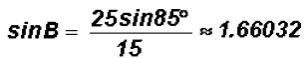However, the result contradicts the fact that the value of sin B is always between1 and 1. Your calculator will give you an ERROR message when you try to solve for angle B.

Given the initial information, the triangle has NO solutions.

Below is a more accurate picture of the given information:Example 4:

Solve the following oblique triangle. Round all calculations to two decimal places.Let's use the Law of Cosines because we do not have at least one side and its opposite angle which is necessary to use the Law of Sines

Solve for Angle A:

Use.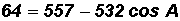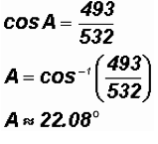Note that 493/532 was not changed to a decimal approximation in the interest of more exact solutions.

There cannot be another solution because the only other quadrant in which cosine is positive is QIV, but there the angles are greater than 180o.

Solve for Angle B:

We'll use the Law of Cosines again. Specifically, we will use.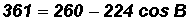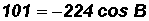Note that101/224 was not turned into a decimal approximation!

Solve for Angle C:

We know that A22.5o and B116.80o

Let's use the fact that the sum of interior angles in a triangle is 180o.

C180o22.08o116.8o = 41.12o

The triangle has the following solutions:

Given a = 8 ft, b = 19 ft, and c = 14 ft,

we found A22.08o, B116.8o, and C41.12o

Example 5:

Solve the oblique triangle pictured below. Round all calculations to two decimal places.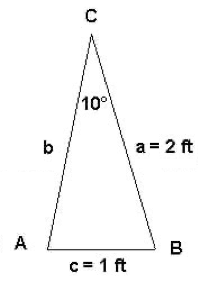Given two sides and one angle opposite one of the sides, we could use the Law of Sines. However, let's instead use the Law of Cosines to show that this law can be used as well.

Solve for Side b:

Let's use.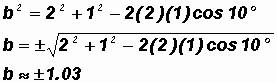Note that the radicand was not changed to a decimal approximation before taking its square root! It is better to keep the numbers as exact as possible for as long as possible.

Disregarding the negative value above (distances are never negative!!!), we get

b1.03 ft

Solve for Angle A:

Let's useusing the UNROUNDED value for Side b.Please note that the fraction above was not turned into a decimal approximation. It was typed into the calculator using appropriate grouping symbols to keep the solution as exact as possible.

A160.27o

There cannot be another angle because the only other quadrant in which cos A is negative is Q III, where the angles are greater than 180o.

Solve for Angle B:

We know that A160.27o and C = 10o.

Let's use the fact that the sum of interior angles in a triangle is 180o.

B180o160.27o10o = 9.73o

The triangle has the following solutions:

Given a = 2 ft, c = 1 ft, and 10o,

we found A160.27o, B9.73o, and b1.03 ft